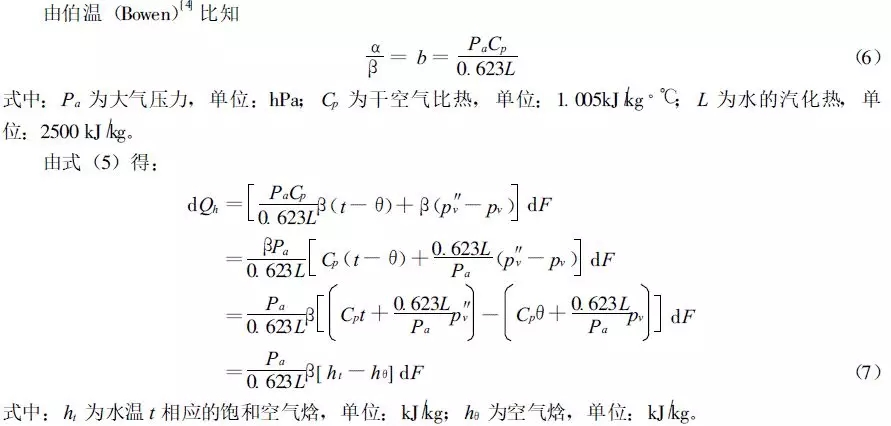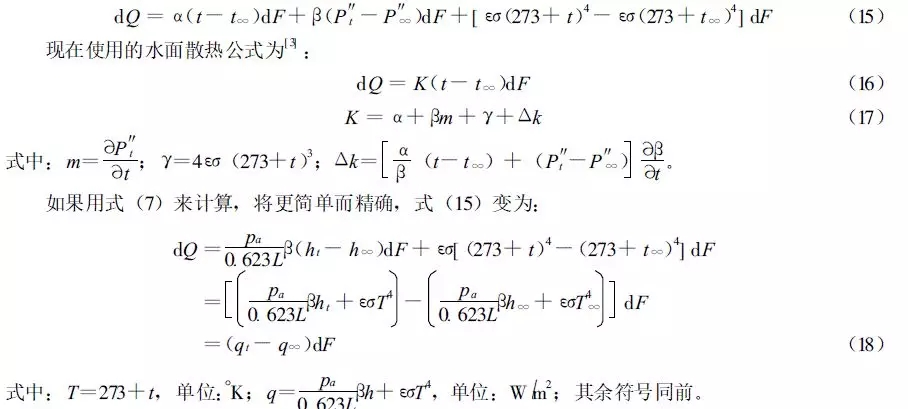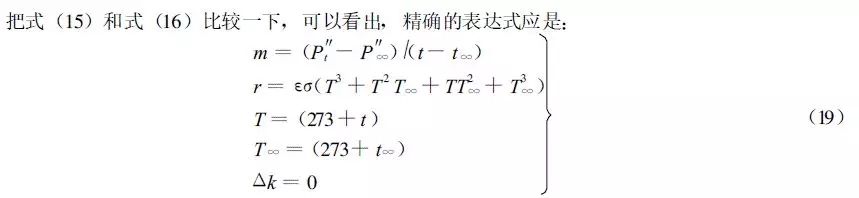# 水面散热的焓差公式及其应用节选

1.对流散热：单位时间、通过水表面dF，水面传给空气的热量dQa，可用下式表示：dQa=α(t-θ)dF (1)

2.蒸发散热：单位时间，通过水表面dF，蒸发的热量dQb，可用下式表示：

dQb= β(p″v-pv)dF (2)

3.辐射散热：辐射散热可按下式计算：dQc=εσ(t+273)4dF(3)

dQ =dQa+dQb+dQc=[α(t-θ)+β(p″v -pv)+εσ(t +273)4] dF (4)

dQh =dQa+dQb =[α(t -θ)+β(p″v-pv)] dF (5)dQb = LdG =LβP(p″v -pv)dF = β(p″v -pv)dF (10)(1)本文给出了把对流散热和蒸发散热合而为一的，以焓差表示的散热公式式(7)。

(2)文中指出，蒸发系数中不应包括水气温差项(t-θ)，应采取式(13)的形式。

(3)文中对式(17)中的K进行了讨论, 认为使用式(18) 将更简单而精确。暖通空调
 电气
 给排水
 高效机房
 建筑智能化
 绿建能耗
 消防
 声学
 垂直交通
 酒店
 医疗
 PMCM
 BIM
 卓展原创